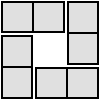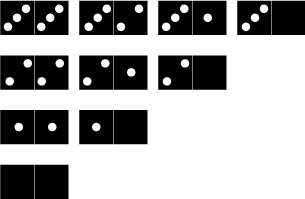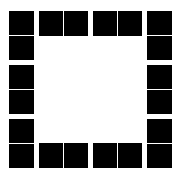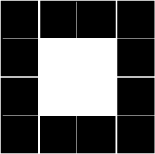#### You may also like### Consecutive Numbers

An investigation involving adding and subtracting sets of consecutive numbers. Lots to find out, lots to explore.### Roll These Dice

Roll two red dice and a green dice. Add the two numbers on the red dice and take away the number on the green. What are all the different possible answers?### 4 Dom

Use these four dominoes to make a square that has the same number of dots on each side.

# Domino Square

## Domino Squares

These are the 'double-3 down' dominoes.Use these dominoes to make this square so that each side has eight dots.### Why do this problem?

Dominoes are a great resource and this problem uses them in an intriguing and curious way. Not only does this activity require logical thinking but it is also an interesting way of practising addition and subtraction, and it provides an opportunity to talk about different ways of recording.

### Possible approach

If you have an interactive whiteboard, you may find our Dominoes Environment useful for this problem.

You could introduce the challenge by laying out ten large dominoes in a square on the floor (it does not matter which dominoes go where). Ask the class to gather round and ask a few questions about the sum of dots on each side so that learners understand how the corner spots are counted in both the horizontal side and the vertical side.

Introduce the problem itself and ask pairs of children to talk for a minute or two about how they might tackle the problem. Share some of their suggestions among the whole group before giving them time to work in their pairs with dominoes. Using real dominoes whenever possible would be advantageous, but you can use this sheet of a standard set of dominoes to be cut out. Squared paper would also be useful for jottings and recording.

As well as talking about the solutions in the plenary, you could focus on how children recorded their solutions. Some may well have just used the dominoes and moved them around as they went but how did they keep track of what they had tried? Some may have jotted down pictures of different arrangements. It would be useful to have a conversation about what ways of recording are most useful in this context.

### Key questions

Tell me what you have done so far.
What do the numbers on this side add to?
What do you need to make eight?

### Possible extension

Use the 'double 4 down' dominoes to make a rectangle with equal numbers of dots on each side. Repeat with 'double 5 down' etc.

What numbers of dominoes can be made into a true square? Explore the numbers that emerge and explain why certain numbers of dominoes cannot be made into a square.

### Possible support

Use real dominoes and sort out the '3 spot down' ones and use them to make a square. Then count the dots on the sides and work on the problem on a 'trial and improvement' basis.

You could start with the 'double 2 down' dominoes making each side add to 16 and using a square like this: